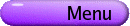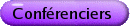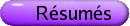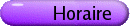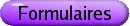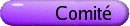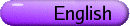Conférenciers pléniers de la SMC[PDF] KEITH DEVLIN, Stanford University How much mathematics can be for all? [PDF] In my book The Math Gene [Basic Books, 2000], I presented an evolutionary argument to show that the basic capacity for mathematical thinking is present in everyone as part of our genetic inheritance. But how much mathematics comes in this way? Is there a point beyond which most people will simply never get it? I believe there is sufficient evidence to suggest that the answer may be yes, and that among those parts of mathematics that can possibly be mastered only by a few is at least one topic taught in the middle school. DAN FREED, University of Texas at Austin Correspondences, K-theory, and loop groups [PDF] Ideas from quantum field theory and string theory continue to influence many fields of mathematics. We consider the Verlinde ring, which originally arose in two dimensional conformal field theory. Let G be a compact Lie group and LG the free loop space of G, viewed as a group by pointwise multiplication. There is a special class of unitary representations of LG in terms of which the Verlinde ring is mathematically defined. In joint work with Michael Hopkins and Constantin Teleman we locate this ring in topology, specifically a twisted version of K-theory. Furthermore, we construct the ring structure-and a Frobenius ring structure-in terms of correspondence diagrams. The talk will include some expository material on correspondences and quantum field theory. ROBERT McCANN, University of Toronto Fluid flow in the semigeostrophic oceans and atmosphere [PDF] The semigeostrophic approximation to Euler's equation is used to caricature the large-scale, long-time evolution of the atmosphere and oceans, and to model such phenomena as the formation and evolution of pressure fronts. Even in 3D, it can be formulated as an active scalar transport model-like the 2D incompressible Euler equations-but with the Hessian of the stream function related to the conserved scalar quantity by a Determinant instead of a Trace. This talk surveys some mathematical developments and open questions concerning this nonlinear model, exposing in particular a family of exact solutions to the equations, representing 2D circulations of an ideal fluid in a elliptical ocean basin. For these special solutions, the fluid pressure and stream function remain quadratic functions of space at each instant in time, whose fluctuations are described by a single degree of freedom Hamiltonian system depending on two conserved parameters: domain eccentricity and the constant value of potential vorticity. These parameters determine the presence or absence of periodic orbits with arbitrarily long periods, fixed points of the dynamics, and aperiodic homoclinic orbits linking hyperbolic saddle points. The energy relative to these parameters selects the frequency and direction in which isobars nutate or precess, as well as the steady circulation direction of the fluctuating flow. Canonically conjugate variables are given, which describing the complete evolution of an elliptical inverse-potential-vorticity patch in dual space. ANDREI OKOUNKOV, Princeton University Enumerative geometry of curves in threefolds [PDF] This will be a review of some mostly conjectural ideas about enumerative geometry of curves of given degree and genus in a smooth projective threefold such as, e.g., projective space. It will be based on my joint work with Davesh Maulik, Nikita Nekrasov, and Rahul Pandharipande. GILLES PISIER, Texas A&M and Paris VI Similarity problems and amenability [PDF] In 1955, Kadison formulated the following conjecture: any bounded homomorphism u : A® B(H), from a C*-algebra into the algebra B(H) of all bounded operators on a Hilbert space H, is similar to a *-homomorphism, i.e., there is an invertible operator x: H® H such that x® xu(x) x-1 satisfies xu(x*) x-1 = (xu(x) x-1)* for all x in A. This conjecture remains unproved, although many partial results are known. We will survey those as well as more recent results on the closely related notion of length of an operator algebra. In particular, we will explain why length equal to 2 characterizes amenable groups or nuclear C*-algebras. KEN RIBET, University of California at Berkeley The modularity of some mod p Galois representations [PDF] I will sketch the main ideas of recent preprints of Khare-Wintenberger and Dieulefait that allow one to establish certain cases of Serre's conjectures on mod p irreducible two-dimensional representations of the Galois group of Q. Recall that the 1994 proof of Fermat's Last Theorem for exponent p associates an elliptic curve E to each putative non-trivial solution of Fermat's equation. The mod p representation E[p] associated to E is incompatible with Serre's conjecture; it cannot be modular. The classical method of Wiles is to prove that E[p] is modular by proving that E is modular. In the new millennium, Khare-Wintenberger and Dieulefait work directly with E[p] and forget where it came from. While the new method may not strike everyone as a simplification of the proof of FLT, it will very likely lead to striking new results.